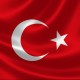Draw a star in C#The following StarPoints method generates the points needed to draw a star and returns them in an array.

// Return PointFs to define a star.
private PointF[] StarPoints(int num_points, Rectangle bounds)
{
// Make room for the points.
PointF[] pts = new PointF[num_points];

double rx = bounds.Width / 2;
double ry = bounds.Height / 2;
double cx = bounds.X + rx;
double cy = bounds.Y + ry;

// Start at the top.
double theta = -Math.PI / 2;
double dtheta = 4 * Math.PI / num_points;
for (int i = 0; i < num_points; i++)
{
pts[i] = new PointF(
(float)(cx + rx * Math.Cos(theta)),
(float)(cy + ry * Math.Sin(theta)));
theta += dtheta;
}

return pts;
}

The method allocates room for the points and then uses a loop to generate them. The variable theta determines the direction of each point with respect to the center of the star. If the star's center has coordinates (cx, cy), then a point on the star has coordinates:

(cx + rx × cos(theta),
cy + ry × sin(theta))

If the code were drawing a regular polygon with num_points sides, then the difference between adjacent theta values would be 2 × π / num_points. In this method, dtheta is twice that value so the lines drawing the star skip polygon points much as you probably do when you draw a five-pointed star. (Note that this only works for stars with an odd number of points.)

The following code shows how the program draws the star.

// Draw a star.
private void Form1_Paint(object sender, PaintEventArgs e)
{
e.Graphics.SmoothingMode = SmoothingMode.AntiAlias;

PointF[] pts = StarPoints(5, this.ClientRectangle);
e.Graphics.DrawPolygon(Pens.Blue, pts);
}This entry was posted in drawing, geometry, graphics, mathematics and tagged , , , , , , , , , , , , , . Bookmark the permalink.

2 Responses to Draw a star in C#

1.Ertuğrul Baran says:

Thanks so much